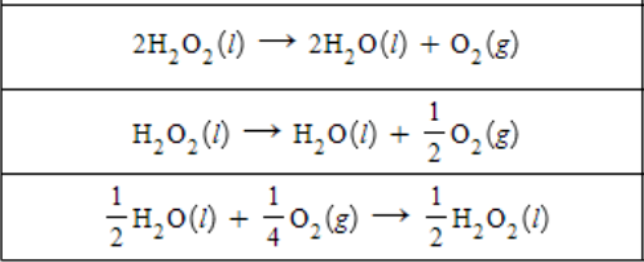# Problem: A chemist measures the enthalpy change ΔH during the following reaction: 2H2O (l) + O2 (g) → 2H2O2 (l)            ΔH = 196. kJ Use this information to calculate ΔH of each reaction. Round each of your answers to the nearest kJ/mol.

###### FREE Expert Solution
86% (415 ratings)
###### FREE Expert Solution

We need to first identify the modifications that were done to the first reaction.

2H2O (l) + O2 (g) → 2H2O2 (l)

86% (415 ratings)###### Problem Details

A chemist measures the enthalpy change ΔH during the following reaction:

2H2O (l) + O2 (g) → 2H2O2 (l)            ΔH = 196. kJ

Use this information to calculate ΔH of each reaction. Round each of your answers to the nearest kJ/mol.What scientific concept do you need to know in order to solve this problem?

Our tutors have indicated that to solve this problem you will need to apply the Hess's Law concept. You can view video lessons to learn Hess's Law. Or if you need more Hess's Law practice, you can also practice Hess's Law practice problems.

What is the difficulty of this problem?

Our tutors rated the difficulty ofA chemist measures the enthalpy change ΔH during the followi...as medium difficulty.

How long does this problem take to solve?

Our expert Chemistry tutor, Dasha took 5 minutes and 39 seconds to solve this problem. You can follow their steps in the video explanation above.

What professor is this problem relevant for?

Based on our data, we think this problem is relevant for Professor Tucker's class at CSU.